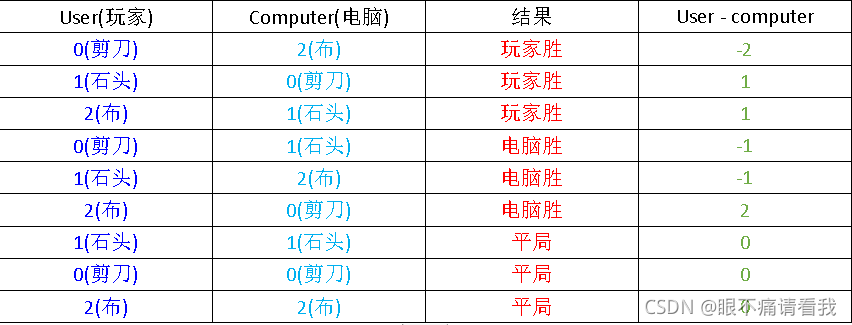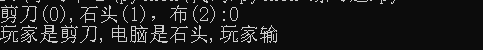﻿ python实现简单石头剪刀布游戏_python_脚本之家

# python实现简单石头剪刀布游戏

更新时间：2021年10月23日 12:27:45   作者：眼不痛请看我```if user == 0 and computer == 0:
print("平局")
elif user == 0 and computer == 1:
print("玩家胜")
elif user == 0 and computer == 2:
print("电脑胜")
elif user == 1 and computer == 0:
print("电脑获胜")
elif user == 1 and computer == 1:
print("平局")
elif user == 1 and computer == 2:
print("玩家胜")
elif user == 2 and computer == 0:
print("玩家胜")
elif user == 2 and computer == 1:
print("电脑胜")
elif user == 2 and computer == 2:
print("平局")

``````1.if user-computer == -2 or user-computer == 1 时，是玩家胜出
2.if user-computer == -1 or user-computer == 2 时，是电脑胜出
3.if user-computer == 0 时，是平局

```

```if user == computer:
print("玩家是%s,电脑是%s,平局"%(usr,com))
elif user - computer == -1 or user - computer == 2:
print("玩家是%s,电脑是%s,玩家输"%(usr,com))
else:
print("玩家是%s,电脑是%s,玩家胜"%(usr,com))

```

```import random
computer = random.randint(0,2)
user = int(input("剪刀(0),石头(1)，布(2):"))
#判断电脑出的是石头，剪刀，还是布
if computer == 0:
com = "剪刀"
elif computer == 1:
com = "石头"
else:
com = "布"
#判断玩家出的石头，剪刀，还是布
if user == 0:
usr = "剪刀"
elif user == 1:
usr = "石头"
else:
usr = "布"
#结果并输出
if user == computer:
print("玩家是%s,电脑是%s,平局"%(usr,com))
elif user - computer == -1 or user - computer == 2:
print("玩家是%s,电脑是%s,玩家输"%(usr,com))
else:
print("玩家是%s,电脑是%s,玩家胜"%(usr,com))

```